# 蒙特卡罗方法入门## 一、概述## 二、π的计算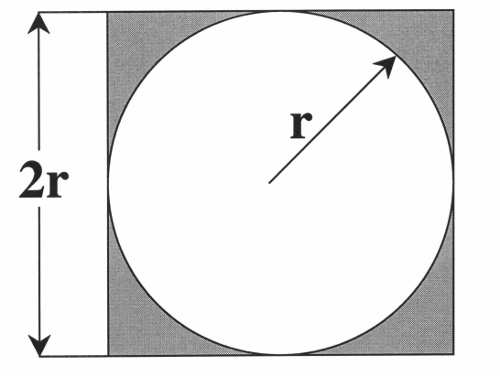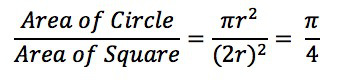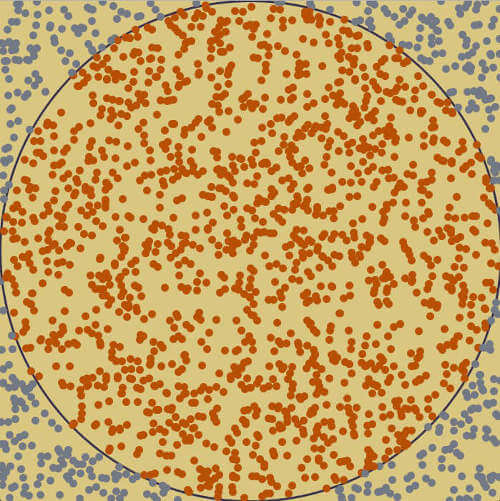## 三、积分的计算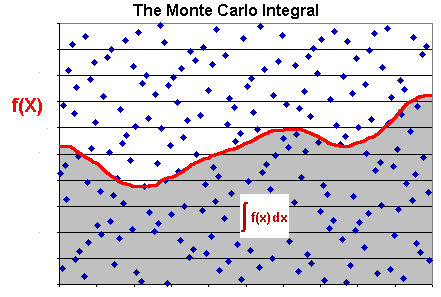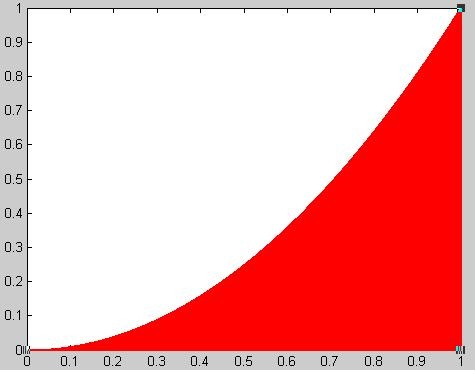## 四、交通堵塞

• 当前速度是 v 。
• 如果前面没车，它在下一秒的速度会提高到 v + 1 ，直到达到规定的最高限速。
• 如果前面有车，距离为d，且 d < v，那么它在下一秒的速度会降低到 d - 1 。
• 此外，司机还会以概率 p 随机减速， 将下一秒的速度降低到 v - 1 。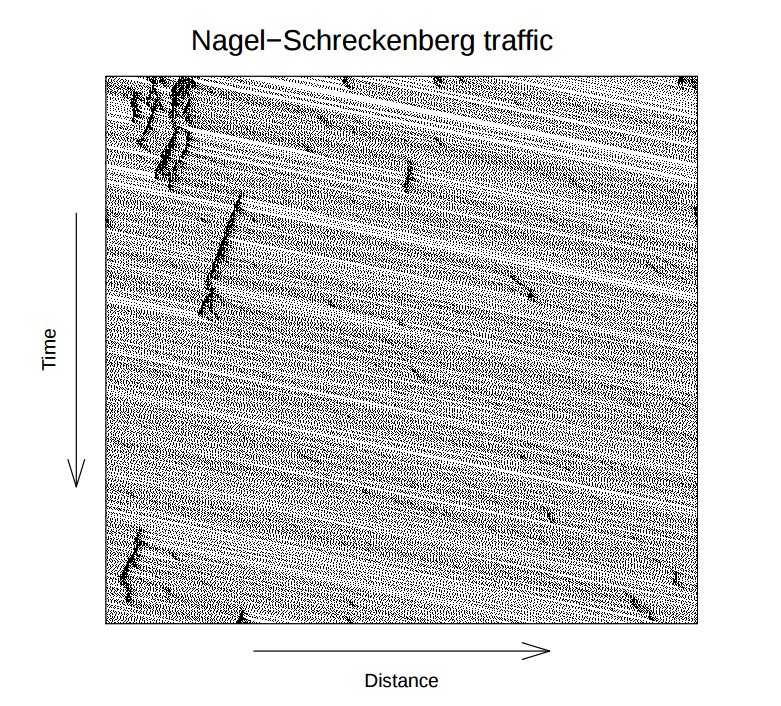## 五、产品厚度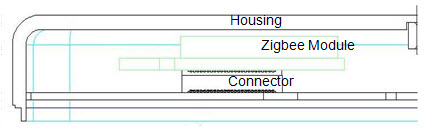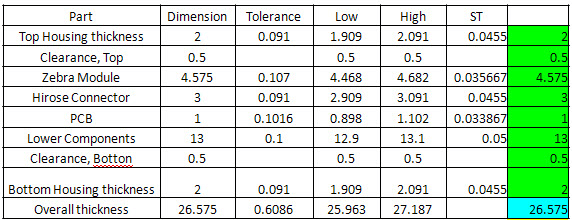## 六、证券市场

• 如果交易冷清，你会以平均价11元，卖出5万股。
• 如果交易活跃，你会以平均价8元，卖出10万股。
• 如果交易温和，你会以平均价10元，卖出7.5万股。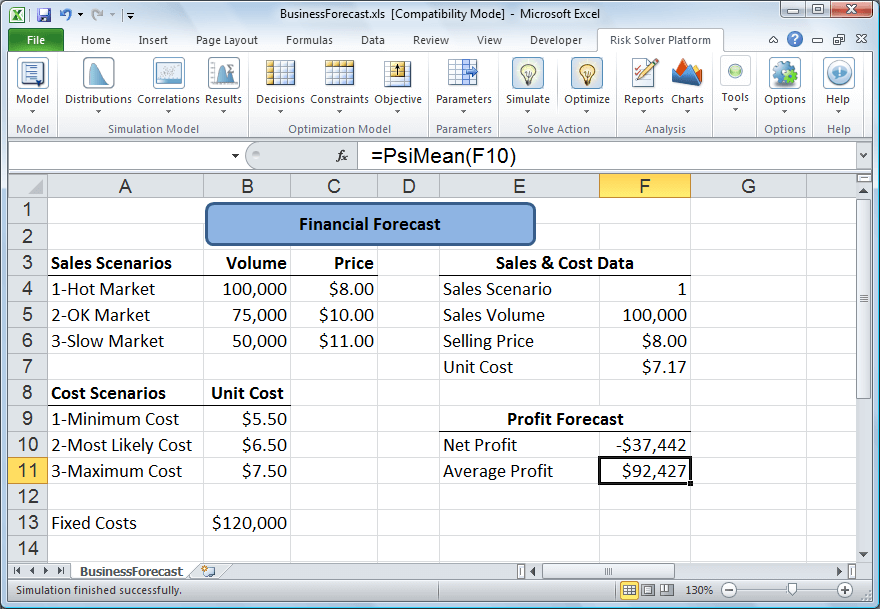（完）

## 留言（34条）

`引用John的发言：`

#-*-coding:utf-8-*-
import random

def countProfit(n):
count = 0;
for i in range(n):
cost = random.uniform(5.5,7.5)
pro = (11 - cost)*5 + (8 - cost)*10 + (10 - cost)*7.5
count += pro;
return count / (3*n)

print countProfit(400)

#!/usr/bin/env python
# -*- coding: utf-8 -*-

from random import random
from math import sqrt

x = y = inn = out = 0.0

for i in range(30000):
x = random()
y = random()
print(x, y)
if (sqrt(x * x + y * y) inn += 1.0
else:
out += 1.0

print(inn / out)

@ooxx：

07年开始订阅你的Blog,到现在，以及将来...每篇必读

`引用芬兰湾的发言：`

`引用jqk6的发言：`

`引用bigTom的发言：`

@ooxx：

@bigTom：

`引用assassindesign的发言：`

@bigTom：

`引用路过不要错过的发言：`

`引用ooxx的发言：`

......
print(inn / out)

`引用ooxx的发言：`

#!/usr/bin/env python
# -*- coding: utf-8 -*-

from random import random
from math import sqrt

x = y = inn = out = 0.0

for i in range(30000):
x = random()
y = random()
print(x, y)
if (sqrt(x * x + y * y)
inn += 1.0
else:
out += 1.0

print(inn / out)

import numpy as np
import random

n = 10000
r = 1.0
data = [random.random()**2 + random.random()**2 for x in range(n)]
num = np.sum(np.array(data) < r**2)

p = 4.0 * num / n
print("pi is roughly: " , p)

`引用ooxx的发言：`

#!/usr/bin/env python
# -*- coding: utf-8 -*-

from random import random
from math import sqrt

x = y = inn = out = 0.0

for i in range(30000):
x = random()
y = random()
print(x, y)
if (sqrt(x * x + y * y)
inn += 1.0
else:
out += 1.0

print(inn / out)

`引用ldb的发言：`

@David Aldrich：

num = 100000;
x = rand(num, 1);
sim_y = rand(num, 1);
eval('y = x.^2;');

area = sum(sim_y

plot_x = 0:0.01:1;
plot_y = plot_x.^2;

plot(x, sim_y, '.');
hold on;
plot(plot_x, plot_y, 'linewidth', 2);
fprintf('area is %d', area);

10万个点，结果是0.3332500

`引用John的发言：`

@John：

«-必填

«-必填，不公开

«-我信任你，不会填写广告链接